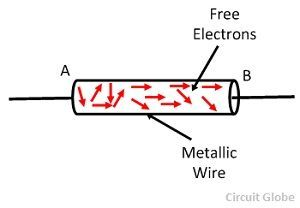# Electric Current

Definition: Electric current is defined as the rate of flow of negative charges of the conductor. In other words, the continuous flow of electrons in an electric circuit is called an electric current.The conducting material consists a large number of free electrons which move from one atom to the other at random.

### Unit of Current

Since the charge is measured in coulombs and time in seconds, so the unit of electric current is coulomb/Sec (C/s) or amperes (A). The amperes is the SI unit of the conductor. The I is the symbolic representation of the current.Thus, a wire is said to carry a current of one ampere when charge flows through it at the rate of one coulomb per second.

When an electrical potential difference is applied across the metallic wire, the loosely attached free electrons start moving towards the positive terminal of the cell shown in the figure below. This continuous flow of electrons constitutes the electrical current. The flow of currents in the wire is from the negative terminal of the cell to the positive terminal through the external circuit.

### Conventional Direction of Flow of Current

According to the electron theory, when the potential difference is applied across the conductor some matter flows through the circuit which constitutes the electric current. It was considered that this matter flows from higher potential to lower potential, i.e. positive terminal to the negative terminal of the cell through the external circuit.This convention of flow of current is so firmly established that it is still in use.Thus, the conventional direction of flow of current is from the positive terminal of the cell to the negative terminal of the cell through the external circuit. The magnitude of flow of current at any section of the conductor is the rate of flow of electrons i.e. charge flowing per second.

Mathematically, it is represented byOn the basis of the flow of electric charge the current is mainly classified into two types, i.e. alternating current and direct current. In direct current, the charges flow through unidirectional whereas in alternating current the charges flows in both the direction.